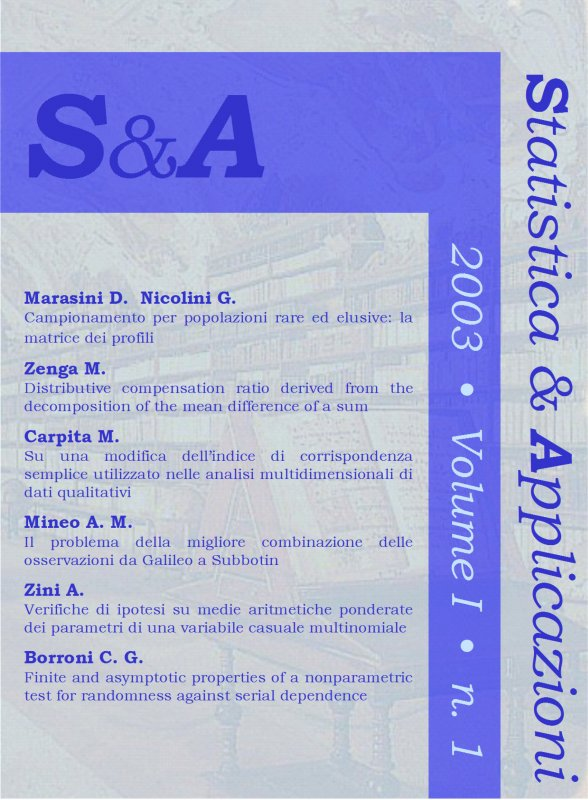Your browser does not support JavaScript!

STATISTICA & APPLICAZIONI - 2003 - 1

digitalDigital issue
 journal STATISTICA & APPLICAZIONI issue 1 - 2003 title STATISTICA & APPLICAZIONI - 2003 - 1 publisher Vita e Pensiero formatDigital issue |Pdf Write a comment for this product

Ebook format Pdf readable on these devices:

• Computer
with Software:
• Android
with App:
• Ibs Tolino
• Kobo
Primo fascicolo del 2003

CONTENTS

Campionamento per popolazioni rare ed elusive: la matrice dei profili
by Donata Marasini, Matteo Nicolini pages: 14
Abstract
We have compared four methods (center sampling, multiple frame, network and adaptive sampling) usually proposed for samples drawn from rare and/or elusive populations, for which we haven’t a frame or, if we have, is incomplete. As the multiple frame can be considered a particular case of center sampling and adaptive a particular case of network, the comparison will be made between center and network. To make a survey on a rare and/or elusive population with L units (that are partially overlapping for the centers and completely separate for the network) and Nobservation units we will draw, at random, some units and define a “profile” for every observation unit in the first method and define a “network” in the second one. In this paper we will show that in theory one method can be considered like the other through a support known as “profile matrix”, which in population has the size NxL. Thus, using this matrix, in the center sampling a set of equal profiles can be called network, while in the network sampling a set of equal profiles can be taken for a network or more than one. This means that once the sample has been drawn, only if a set of equal profiles characterizes a single network will the numerator of the estimators proposed for the two methods will then coincide.

Keywords: adaptive sampling, center sampling, multiple frame, network.
Distributive Compensation Ratio Derived from the Decomposition of the Mean Difference of a Sum
by Michele Zenga pages: 10
Abstract
In this paper a distributive compensation index, based on the decomposition of the mean difference of a sum Y of the k variates X1, … , Xi, … , Xk , has been proposed. The index takes value 0 if among the k variates there is no-compensation, which means that the k variates are uniformly ranked. The index is equal to 1 if among the k variates there is maximum compensation, which means that the sum X1 + … + Xi + … + Xk is constantly equal to the mean μ(Y). It has been also shown that if the k variates assume the integer values 1, 2, … , N, then the index is equal to: 1 – 3G(Y), where G(Y) is Gini’s concentration index evaluated on the sum.

Keywords: Mean difference, Uniform ranking, Co-graduation, Maximum Compensation, Compensation term, Compensation ratio.
Su una modifica dell’indice di corrispondenza semplice utilizzato nelle analisi multidimensionali di dati qualitativi
by Maurizio Carpita pages: 18
Abstract
In multidimensional analysis for qualitative data a similarity and/or dissimilarity matrix is commonly used. When the analysis concerns the presence-absence of each of p attributes for pairs of objects, often the generic (i , j)-element of this matrix is the value of the simple matching coefficient S computed on the positive-negative matches and the mismatches associated with the p binary attributes for the (i, j)-objects. But there are situations in which it can be useful to adjust the classical S index in order to take into account, as well as the usual positive-negative matches, the mismatches too.

Keywords: Multivariate Analysis, Similarity, Simple Matching Coefficient.
Il problema della migliore combinazione delle osservazioni da Galileo a Subbotin
by Angelo M. Mineo pages: 20
Abstract
In this paper it is sketched a brief historical picture of the proposals presented in the old statistical literature on the methods to combine opportunely observations with random errors. Then it has been done particular reference to the exposure of the procedure followed by Gauss to derive the so called normal distribution and that followed by Subbotin to derive a generalization of the normal distribution, that with a different parametrization coincides with the normal of order p distribution, also known as general error distribution.

Keywords: Random errors, Normal distribution, Subbotin.
Verifiche d’ipotesi su medie aritmetiche ponderate dei parametri di una variabile casuale multinomiale
by Alessandro Zini pages: 18
Abstract
In this work the hypothesis testing problem that a weighted mean of the probabilities of a multinomial distribution does not exceed a target value, with unilateral alterative will be studyed. Two kinds of tests will be considered: an “analigical” test and a likelihood ratio-based test. The former, though not asymptotically similar, turns out to be useful in finding the sample size when both error probabilities are fixed. The latter, which is asymptotically optimal, is good when the sample size is not less than 50.

Keywords: Distribuzioni esatte , Rapporto di verosimiglianza, Stimatore BAN.
Finite and Asymptotic Properties of a Nonparametric Test for Randomness against Serial Dependence1
by Claudio Giovanni Borroni pages: 15
Abstract
A very important problem in time series analysis is testing for randomness against serial dependence. Classical parametric methods, commonly based on the autocorrelation coefficient, can be misleading when the underlying distributional assumptions are not fulfilled. In this paper the use of a nonparametric measure of serial dependence, based on Gini's cograduation index, is discussed. More specifically, the finite and asymptotic properties of this test-statistic are discussed; the test is then compared with its competitors via asymptotic relative efficiency.

Keywords: Nonparametric tests, Serial dependence, Gini's cograduation index, U-statistics, Linear serial rank statistics.